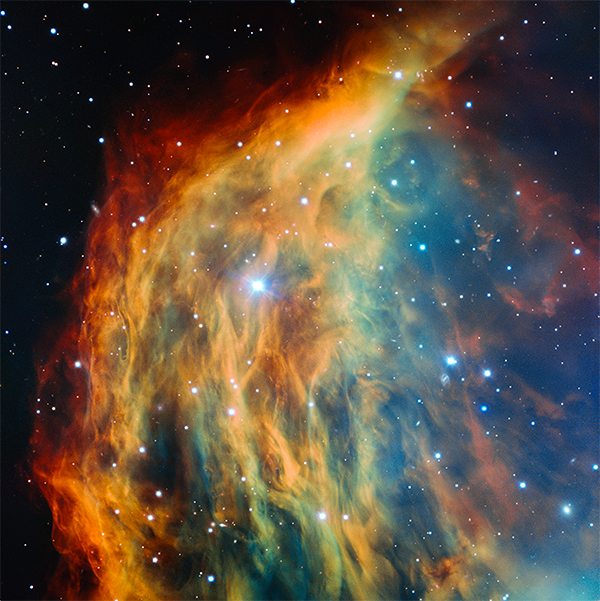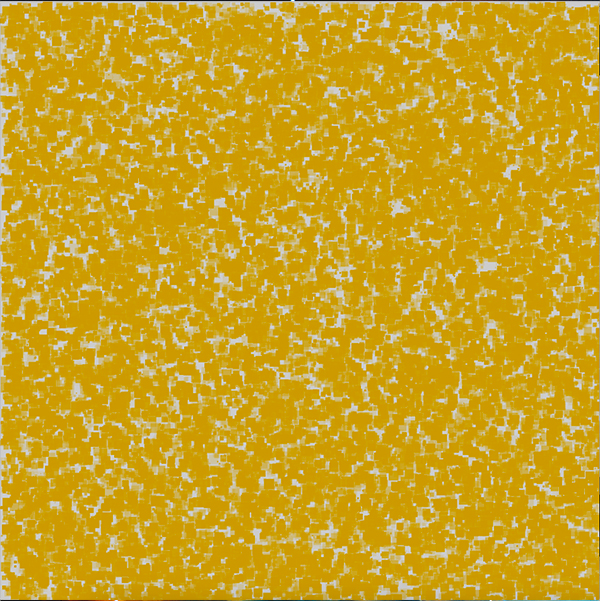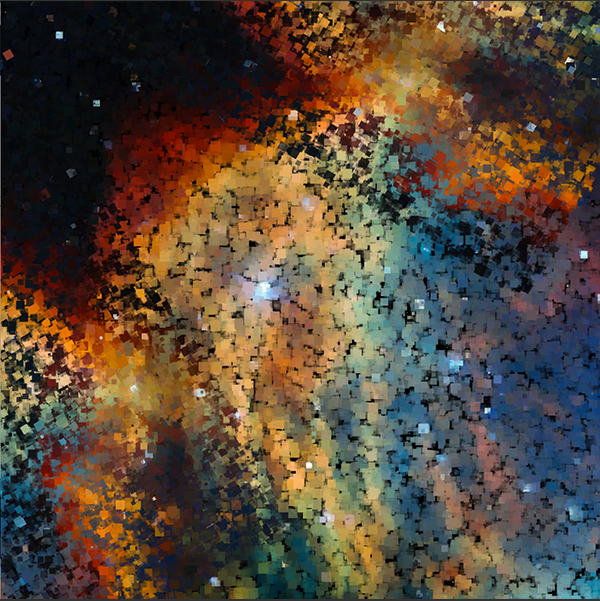PImage, and color in Processing

I wanted to explore little bit with the PImage object in Processing. Below, are some variations of studies using PImage object.

//Load an image and then display it
PImage img;
void setup(){
size(950, 950,P3D);
}

void draw(){
image(img, 0, 0);
}Now. let’s think of a simple animation. Maybe, we’ll start with some random squares in the screen, we’ll give them a color, and we’ll define some opacity.

void setup(){
size(950, 950,P3D);
}

void draw(){
float x = random(width);
float y = random(height);
float size = random(1,16);
color c = color(204, 153, 0);
fill(c, random(255));
noStroke();
rect(x,y,size,size);
}

Also, you can put a loop in the drawing function. The rectangles will populate faster the stage.

for (int i =0; i<20; i++){
float x = random(width);
float y = random(height);
float size = random(1,16);
color c = color(204, 153, 0);
fill(c, random(255));
noStroke();
rect(x,y,size,size);
}Why not render the image color information into the ractangles. See code below

PImage img;
void setup(){
size(950, 950,P3D);
background(0);
}
void draw(){
for (int i =0; i<100; i++){
float x = random(width);
float y = random(height);
float size = random(1,16);
color c = img.get(int(x),int(y));//store the color from the image
fill(c, random(255));//color the rectangles with the image colors
noStroke();
rect(x,y,size,size);
}
if (frameCount % 700 == 0) {
background(255);//reset the stage
}
}